Skip to main content

# Study on the influence of slope height and angle on the factor of safety and shape of failure of slopes based on strength reduction method of analysis

## Abstract

### Background

Decreasing slope angle and slope height increases the slope factor of safety and can change the shape of likely slope failure. The increase in the factor of safety is at different rates, which can depend on soil type and slope geometry. Understanding the relationship between the slope height and angle decrease with the increase in factor of safety is vital to implement an efficient method of increasing factor of safety for slope stability problems. In addition, the shape of slope failure has to be observed thoroughly, not to increase the sliding mass of soil for a likely slope failure, even though the factor of safety has increased.

### Results

Three homogeneous slopes of different soil characteristics were analyzed several times by changing the slope height and angle to determine the factor of safety. The shape of failure was also observed and recorded for each slope height and angle decrease. The analysis results indicated that decreasing slope angle increases the factor of safety nearly linearly while a decrease in height increases the factor of safety at a parabolic rate. Slope height decrease increased the factor of safety at a higher rate for the clay soil while slope angle decrease increased factor of safety at a higher rate for sandy soil compared to the other types of soils considered. The toe slide was observed in clayey and sandy clay soils at higher slopes while the base slide was observed at slopes whose height is less than 2 m. The slope slide was dominant on sandy soil at different slope heights and slope angles.

### Conclusions

While the factor of safety of slopes had increased with slope height and angle decrease, the rate of increases and thus the efficiencies are different which depended on the type of soil and geometry of slope. The shape of failure also changed which might increase the sliding mass of soil. This can be risky if slope failure occurs due to unforeseen events. Using the slope height and angle decrease methods for slope stabilization should be thoroughly investigated to choose the most efficient method and also should be checked not to increase the sliding mass of soil for a possible slope failure.

## Background

Slope stability problems are quite common and widespread in many civil engineering projects. Slope stability problems are particularly encountered in large and important projects including dams, highways, and tunnels . Slope stability problems can cause very devastating social and economical losses . Cuts, fills, dams, and road embankments require slope stability analysis for the safety of humans and property. Adequate inspection and solution should be provided for slopes to alleviate potentially catastrophic failure. There are different techniques for stabilizing slopes. Decreasing slope height and angle are geometric methods of ensuring the safety of slopes by minimizing the risk of failure.

### Slope stability analysis

Slope stability analysis is one of the most important subjects in geotechnical engineering [3, 4]. Slope failures depend on the slope geometry, soil type, soil stratification, groundwater, and seepage. Calculating factor of safety is the general usual procedure in slope stability analysis . There are different methods of slope stability analysis and calculating factor of safety. In limit equilibrium, Factor of Safety (FoS) is calculated as the ratio of shear strength of the soil (τf) to  the mobilized shear (τm) at failure surface as given in Eq. 1.

$$FoS=\frac{\tau f}{\tau m}$$
(1)

Different authors developed different techniques of calculating factor of safety including computer-based finite element analysis techniques. At present time, no single one of the analysis methods is preferred over others and the reliability of any solution is completely left to the engineer in charge . On hand calculations, the usual problem engineers’ face is to locate the critical slip surface. There are some procedures recommended by different scholars to locate the critical slip surface. Some of the early works include grid counter methods , Siegel’s method for non-homogenous slopes with a weak layer , and Carter’s method for non-circular slips using Fibonacci sequence . Recent developments include genetic algorithms , simple genetic algorithm , leapfrog algorithm , annealing algorithm , and more. However, many engineers usually prefer to use their experience to locate the critical surface and precede trial and error procedure until the minimum critical surface is determined.

### Finite element analysis

Nowadays, with the aid of software packages, slope stability analysis is carried out much more easily through mathematical modeling. Mathematical modeling has been used in a wide range of disciplines including social science, medicine, and engineering to predict some natural behaviors [13,14,15,16]. The use of mathematical modeling in civil engineering is currently prominent in almost all design and analysis works.

In finite element analysis of slopes through numerical modeling, prior assumption of the critical surface is not necessary, unlike the hand calculation. The finite element method of calculating the factor of safety is based on the strength reduction method. In this method, also called the phi-c-reduction method, the strength parameters of the soil are simultaneously reduced until failure occurs. The factor of safety is then calculated as the ratio between the actual strength parameters, C and ϕ, of the soil and the critical parameters. If shear strength parameters at failure are Cr and ϕr, the factor of safety, (FoS) is defined as ;

$$FoS=\frac{tan\varphi}{tan\varphi r}=\frac{C}{Cr}$$
(2)

The method has been used by different authors and is incorporated in different commercial softwares of slope analysis [18,19,20]. The phi-c-reduction method is based on the Mohr-Coulomb failure criterion. The definition of the factor of safety in the strength reduction method is exactly the same as in the limit equilibrium method . Researches indicate that the results of limit equilibrium and finite element analysis are the same . Generally, however, limit equilibrium methods give slightly conservative results .

The effect of soil strength parameters on the factor of safety and location of slip surface has been studied a number of times. Lin and Cao discussed the relation between soil unit weight, cohesion, angle of friction, and slope height and their effect on the location of the critical surface . The paper discussed that as λ (the ratio of cohesion to the product of unit weight (γ), slope height (H), and tan of the angle of friction (φ)) is constant; the location of the critical surface remains the same. The paper indicated greater lambda value indicates a deeper failure surface.

$$\lambda =\frac{C}{\gamma Htan\varphi}$$
(3)

The American Society of Civil Engineers has established principles of sustainability in Policy 418. The policy calls civil engineers to “Do the Right Project; Do the Project Right; Perform Life Cycle Assessment from Planning to Reuse; Use Resources Wisely; Plan for Resiliency and Validate Application of Principles.” .  This emphasizes the requirement for thorough study of safety of projects. Slope stability can be improved by changing the slope geometry and adding support structures to reinforce the slope. The factor of safety of slopes can be increased by decreasing the slope angle and/or slope height. However, the increase in the factor of safety in slopes by decreasing slope angle and/or slope height can depend on the type of soil. Moreover, the efficiency in increasing the factor of safety using either of the methods can depend on the type of soil. For some types of soils, decreasing slope angle may be more efficient than decreasing the slope height and for other types of soils, the reverse may hold true. In addition, the increase in factor of safety using slope angle and/or slope height decrease can change the failure surface of possible slope failure thereby changing the failure mass. This might increase the failing mass of soil in a possible slope failure due to unforeseen events. Thus, thorough understanding and analysis is required to use an efficient and adequate method of slope stability procedure to ensure safety. This paper discusses the influence of slope height and angle on the factor of safety of slope and mode of slope failure based on strength reduction method.

## Methods

A slope stability analysis typically considers slope geometry, soil mechanical properties, and groundwater conditions to determine the factor of safety . This emphasizes the importance of slope geometry in the determination of slope factor of safety. Slope stability analysis methods can be broadly classified as limit equilibrium and finite element analysis. Limit equilibrium analysis procedure follows locating a trial slip surface and estimate the factor of safety comparing the resisting force to the driving force. It is a trial and error procedure where slip surfaces are assumed until the minimum factor of safety is reached. On the other hand, finite element analysis does not require trial critical surfaces to calculate factor of safety. Using phi-c reduction methods, the factor of safety can be easily determined. In this study, a finite element-based software, Plaxis-2d version 8 is used to calculate factor of safety of different slope models whose soil height and slope are varied systematically to study their effect on the factor of safety and critical surface. The estimated factor of safety is studied in relation to the parameters.

Numerical analysis results are usually displayed in the form of figures and tables. The power of the shape-preserving interpolation technique used in the application software is an important parameter for achieving smooth visual or graphical representation of data . In computer-aided designs, geometric shapes are related to the mathematical representations that satisfy approximation and interpolation properties of curves and surfaces . Shape preservation is very important in geometric modeling and visualization [28, 29]. Subdivision scheme is the technique of generating curves and surfaces by iterative refinement of initial control polygon/mesh accordingly some refinement rules.

### Types of slope failure

Slope failure can be translational slide, rotational slide, and flow. The rotational slide is common in fine-grained and homogeneous soils. The rotational slide has three types as shown in Fig. 1. The base slide occurs when slope failures occur over an arc inclosing the whole slope. The toe slide occurs when the failure arch passes through the toe of the slope. The slope slide occurs when the failure occurs on the slope.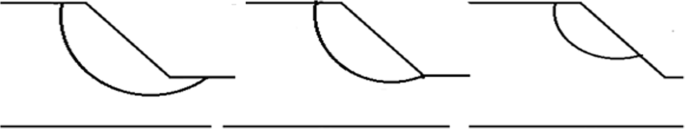Fig. 1

### Slope stabilization methods

There are different methods of slope stabilization. Broms and Wong  identified the different methods as geometric and structural. Geometric methods involve decreasing slope angle from steep to a gentler slope to increase the stability of slope. In addition, the angle can also be supported by grass bonding together with the soil. Decreasing the height of slope can also be used so that the gravitation driving load will be decreased. One cause of slope failure is saturation and pore water pressure build-up in the subsoil. The chances of building up pore water pressure and saturation of subsoil can be minimized by providing proper drainage. This method is used in combination with geometric methods. The other method of slope stabilizing is retaining Structure. This method uses retaining structure to resist the downward forces of the soil mass.

### Numerical modeling and soil property

In this study, the three types of slope soils are modeled in Plaxis. The three types of soils are sandy-clay, clayey, and sandy soils as given in Table 1. Those types of soils are considered to model different types of soil shear strengths.

Three slope models are created in Plaxis-2D. The first model is where the slope height is decreased gradually from 12 m in height to 1m in height at 1 m interval. The second model is where the slope angle is decreased systematically by increasing the horizontal length keeping the height constant of 12 m. The third model is where the slope height and angle are simultaneously decreased. The models are given in Figs. 2, 3, and 4, respectively.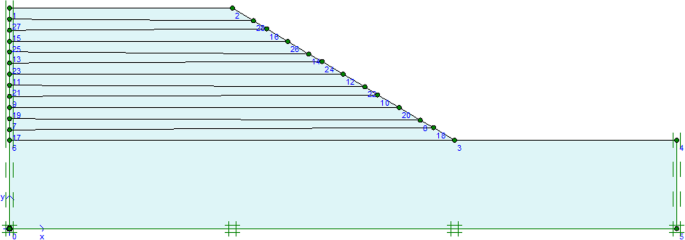Fig. 2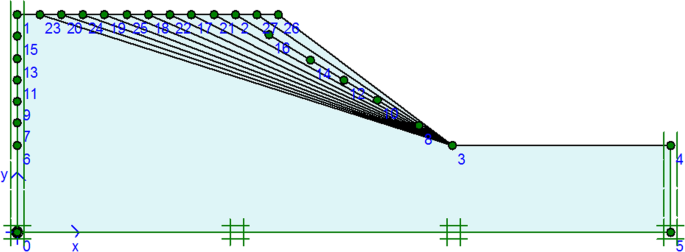Fig. 3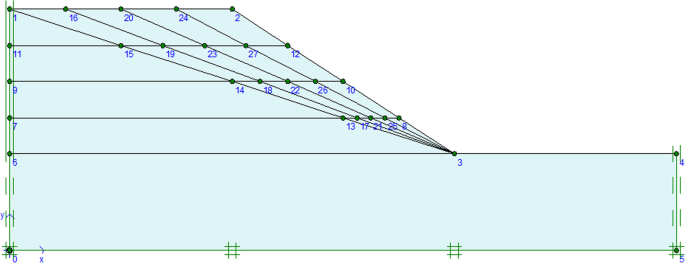Fig. 4

A plane strain model with a triangular 15-node medium coarse mesh element is used in the analysis as shown in Fig. 5. The water table is taken below the bottom level considered.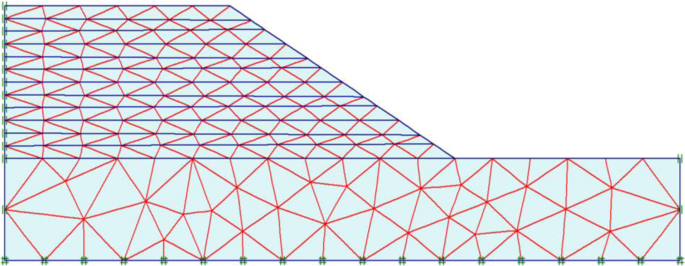Fig. 5

## Results

### Deformation

The mode of slope failure depends on the type of soil and slope geometry among other factors. For a slope of 12 m high and 3V:5H slope, the critical surface passes through the toe of the slope for the clayey and sandy clay soils while for the sandy soil, it passes through the slope as shown in Figs. 6, 7, and 8, respectively. The modes of slope failure for the three types of soils considered changes with the height of the slopes. The different modes of the slope failures as the slope height and angle change are summarized in Tables 2 and 3.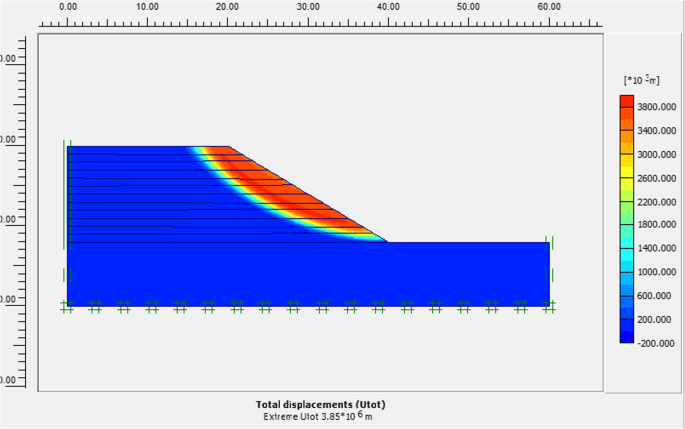Fig. 6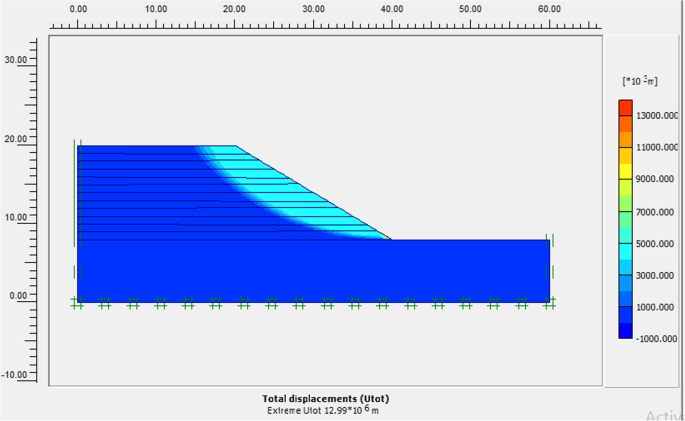Fig. 7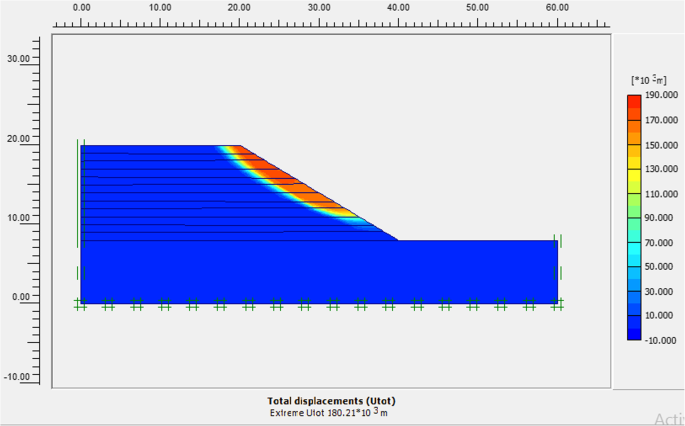Fig. 8

### Slope height and factor of safety

The first analysis to determine the factor of safety is done by decreasing slope height from 12 m to 1 m gradually at 1m interval keeping other parameters constant.

The factor of safety increases as the slope height decreases as shown in Table 4 for the three types of soils considered. The correlation coefficient r indicates a strong opposite relationship between slope height and factor of safety.

### Slope angle and factor of safety

The second analysis procedure is done by varying the slope angle gradually keeping the height of the slope, the soil parameters, and the water level constant. The factor of safety increases as the slope angle decreases as shown in Table 5.

### Slope height and angle

The third analysis model is carried out by decreasing the slope height and angle simultaneously keeping the soil parameter constant. Only one type of soil, sandy clay soil, is considered in this analysis. The calculated factor of safety for different slope heights is given in Table 6.

## Discussion

### Effect of mesh arrangement and calculation steps

The mesh arrangement (Fig. 9) and calculation steps can induce small differences in the calculated results of the factor of safety. For example, comparing the height model and slope mode for the sandy clay soil, the factors of safety calculated are 1.1524 and 1.1466, respectively, which are 0.5% different due to mesh arrangement as shown in the figure below, though all the geometric and soil parameters are similar.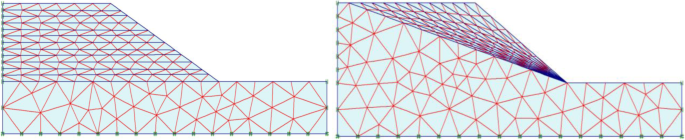Fig. 9

Similarly, the additional steps required to complete the calculation can cause small differences in the calculated factor of safety. For example, for sandy clay soil slope of height 12 m and slope angle 3V: 5H, the factor of safety calculated using 200 additional steps is 1.1466 while using 30 additional steps is 1.1447 which has a 0.17% deviation from the earlier calculation.

### The influence of slope height and slope angle on failure mode of slopes

As described above, the slope failure mode can be base slide, toe slide, and slope slide. The mode of failure depends on the type of soil and slope geometry. As given in Tables 4 and 5, the failure mode of the slopes changes with slope height and slope angle.

For clay and sandy clay soils, the dominant type of slope failure is toe slide. For sandy soil, the dominant mode of failure is the slope slide. At lower heights, i.e., less than 2m height, failure mode in sandy clay and clay soils tends to be base slide while on sandy soil and the failure mode tends to be toe slide.

At steep slopes, sandy clay soils tend to fail under slope slide type of failure, while at gentle slopes, the failure mode changes to toe slide. For clayey soils, the dominant mode of failure is toe slide. The base slide occurs for slopes of an angle less than 18°. The mode of failure on sandy soil is slope failure. The base failure occurs at a steeper slope, i.e., slope angle equal to 36.87°.

### The influence of slope height

The decrease in slope height at the initial stage tends to increase the factor of safety at a lower rate. However, the increase in factor of safety is at a higher rate for height decreases less than 3 meters (Fig. 10).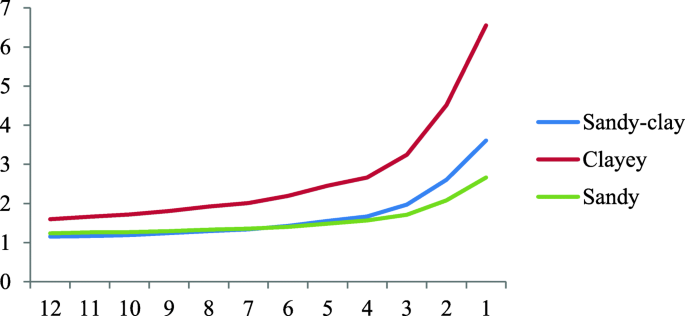Fig. 10

As shown in the figure above, for slope height of 12 m to 3 m, the slope of the line is fairly constant and the correlation analysis indicates there is a strong opposite linear relation between the slope height and factor of safety, i.e, r=−0.9918. At the height of 3 m, there is a sudden change in slope of the graph slope height versus the factor of safety. The correlation analysis from 3 m in height to 1 m in height gives r =−0.96018. The overall correlation coefficient is −0.82872 that indicates that there is a lower rate of decrease in factor of safety in the early decreases of height, i.e., 12 m to 3 m of height. After 3 m of slope height, the factor of safety increases at a higher rate.

### The influence of slope angle

The decrease in slope increases the factor of safety almost linearly. As shown in Fig. 11, the relation between slope angle and factor of safety is approximately linear. The correlation coefficient between slope angle and factor of safety is −0.9761. The correlation coefficient indicates a strong and opposite relation between slope angle and factor of safety for the three types of soils considered.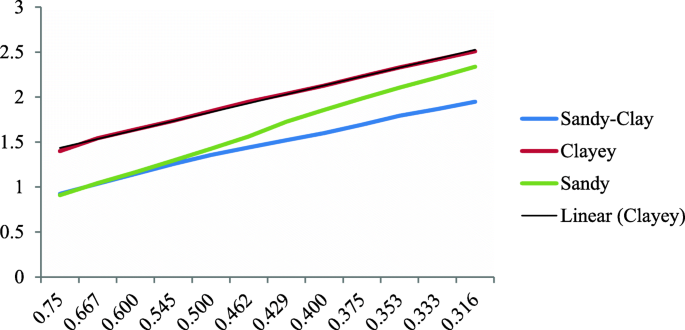Fig. 11

### Slope height and slope angle

Slope height and slope angle can be optimized to maximize the slope factor of safety. The influence of decreasing slope height and angle simultaneously is studied by calculating the factor of safety simultaneously as given in Table 6.

As shown in Fig. 12, for slope heights less than 3m, decreasing slope angle increases the factor of safety at a higher rate than for slopes higher than 3 m. The slope of the graph of slope angle versus the factor of safety for 3-m high slope is 3 while for 6-m, 9-m, and 12-m high slopes, it is 2.69, 2.59, and 2.5, respectively. Thus, for smaller height slopes, decreasing the slope angle increases the factor of safety at a higher rate.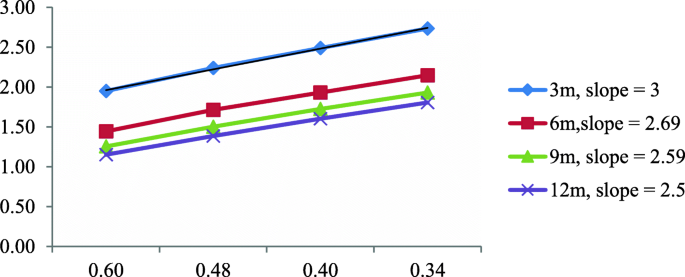Fig. 12

Similarly, at smaller slopes, decreasing the height increases the factor of safety at a higher rate compared to stepper slopes. Figure 13 shows the variation of the factor of safety with height at different slope angles.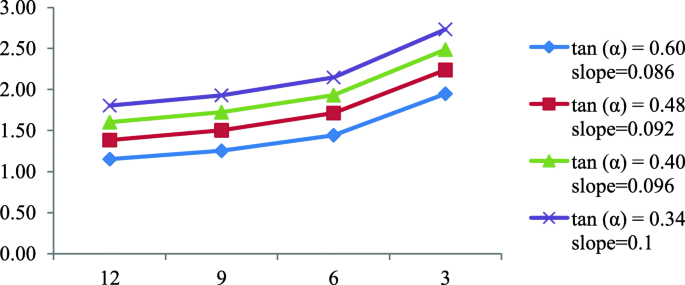Fig. 13

## Conclusion

Based on the analysis results, the failure mode for the three types of soils is found to depend on slope height and angle. For clay and sandy clay soils, the dominant type of slope failure is toe slide. For sandy soil, the dominant mode of failure is the slope slide. At lower heights, i.e., less than 2m height, the failure mode in sandy clay and clay soils tends to be base slide while on sandy soil, the failure mode tends to be toe slide. At steep slopes, sandy clay soils tend to fail under slope slide type of failure, while at gentle slopes, the failure mode changes to toe slide. For clayey soils, the dominant mode of failure is toe slide. The base slide occurs for slopes of an angle less than 18°. The mode of failure on sandy soil is slope failure. The base failure occurs at a steeper slope, i.e., slope angle equal to 36.87°.

Decreasing slope angle increases the factor of safety of slopes nearly linearly while decreasing slope height increases the factor of safety at different rates. For a particular slope, decreasing the slope angle will increase the factor of safety more efficiently than decreasing the slope while for another slope, decreasing height is more efficient. Understanding the failure mode and influence of geometric alteration on slope factor of safety is useful to adopt the most efficient method to increase the stability of slopes.

## Availability of data and materials

All the data is available in the manuscript.

FoS:

Factor of safety

## References

1. 1.

Pourkhosravani, Amin and Kalantari, Behzad. s.l. A review of current methods for slope stability evaluation: Electron J Geotechnical Eng, 2011, Vol. 16, pp. 1245-1254.

2. 2.

Halder A, Nandi S, Bandyopadhyay K (2020) A comparative study on slope stability analysis by different approaches. Geotechnical Characterization and Modelling. Lecture Notes in Civil Engineering, vol. 85. Springer, Singapore. https://doi.org/10.1007/978-981-15-6086-6_23.

3. 3.

Morgenstern NR (1992) The evaluation of slope stability— a 25 year perspective. ASCE Special Geotechnical Publication No 31. https://doi.org/10.1016/0148-9062(93)93166-U.

4. 4.

Yang XL, Huang F (2009) Slope stability analysis considering joined influences of nonlinearity and dilation, vol. 16. School of Civil and Architectural Engineering, Central South University, Changsha, pp. 292–296. https://doi.org/10.1007/s11771−009−0050−2

5. 5.

Albataineh N (2006) Slope stability analysis using 2D and 3D methods. The University of Akron, https://etd.ohiolink.edu/apexprod/rws_etd/send_file/send?accession=akron1153719372&disposition=attachment

6. 6.

Boutrup, E. and Lovell, C. s.l. Searching techniques in slope stability analysis: Eng Geol, 1980, Vol. 16, 1, pp. 51-61.

7. 7.

Siegel RA (1975) Computer analysis of general slope stability problems. Joint Highway Research Project, Indiana Department of Transportation and Purdue University, West Lafayette, Indiana, 1975, Vols. Publication FHWA/IN/JHRP-75/08. https://doi.org/10.5703/1288284313895.

8. 8.

Carter RK (1971) Computer oriented slope stability analysis by method of slices. Thesis, Purdue University, West Lafayette

9. 9.

Goh, A. T. Genetic algorithm search for critical slip surface in multiple-wedge stability analysis. s.l. : Can Geotechnical J, 1999. Vol. 36, 2, pp. 382-391.

10. 10.

Zolfaghari, A. R., Heath, A. C. and McCombie, P. F. Simple genetic algorithm search for critical non-circular failure surface in slope stability analysis. s.l. : Comput Geotechnics, 2005. Vol. 32, 3, pp. 139-152.

11. 11.

Bolton, H., Heymann, G. and Groenwold, A. Global search for critical failure surface in slope stability analysis. s.l. : Eng Optimization, 2003. Vol. 35, 1, pp. 51-65.

12. 12.

Cheng, Y. Location of critical failure surface and some further studies on slope stability analysis. s.l. : Comput Geotechnics, 2003. Vol. 30, 3, pp. 255-267.

13. 13.

Souna, Fethi, Lakmeche, Abdelkader and Djilali, Salih. The effect of the defensive strategy taken by the prey on predator–prey interaction. J Appl Math Comput, 2020, Vol. 64, pp. 665–690. https://doi.org/https://doi.org/10.1007/s12190-020-01373-0.

14. 14.

Djilali, Salih, et al. Turing-Hopf bifurcation in a diffusive mussel-algae model with time-fractional-order derivative. s.l. : Chaos Solitons Fractals, 2020, Vol. 138(C). DOI: https://doi.org/10.1016/j.chaos.2020.109954.

15. 15.

Djilali, Salih, Touaoula, Tarik Mohammed and Miri, Sofiane El-Hadi. A heroin epidemic model: very general non linearincidence, treat-age, and global stability. s.l. : Acta Applicandae Mathematicae, 2017, Vol. 152. DOI https://doi.org/10.1007/s10440-017-0117-2, 1, 194.

16. 16.

Boudjema, Ismail and Djilali, Salih. Turing-Hopf bifurcation in Gauss-type model with cross diffusion and its application. s.l. : Nonlinear Stud, 2018, Vol. 25, 3, pp. 665-687.

17. 17.

Indra NH, Helmut F (2011) Slope stability analysis of unsaturated soil with fully coupled flow-deformation analysis. IAMG publication Salzburg, Austria

18. 18.

Dawson, E. M., Roth, W. H. and Drescher, A. Slope stability analysis by strength reduction, s.l. : Géotechnique, 1999, Vol. 49(6), pp. 835-840.

19. 19.

Griffiths, D. and Lane, P. Slope stability analysis by finite elements. s.l. : Geotechnique, 1999. Vol. 49, 3, pp. 387-403.

20. 20.

PLAXIS. Delfit University of Technology & PLAXIS b.v. Plaxis version 8 Dynamic Manual. A.A. Balkema Publishers, 2002.

21. 21.

Azadmanesh, M. and Arafati, N. A Comparison on Slope Stability Analysis of Aydoghmoosh Earth Dam by limit equilibrium, finite element and finite difference methods. s.l. : IJCEBM, 2012I. pp. 115-124.

22. 22.

Khabbaz, H. F. and Behzad, N. C. Finite element methods against limit equilibrium approaches for slope stability analysis. s.l. : Geomechanical Society and New Zealand Geotechnical Society, 2012.

23. 23.

Lin, H. and Cao, P. Potential slip surfaces of slope with strength parameters. s.l. : Adv Mater Res, 2011. Vol. 243, pp. 3315-3318.

24. 24.

ASCE (American Society of Civil Engineers) (2018) Policy Statement 418 – the Role of the Civil Engineer in Sustainable Development. ASCE. https://www.asce.org/issues-and-advocacy/public-policy/policy-statement-418---the-role-of-the-civil-engineer-in-sustainabledevelopment/.

25. 25.

Shepheard, Casey J., et al. Analysis of design choices for a slope stability scenario in the humid tropics. ES1, s.l. : Engineering Sustainability, 2018, Vol. 171.

26. 26.

Harim, Noor Adilla, et al. s.l. Positivity preserving interpolation by using rational quartic spline. : AIMS Math, Vol. 5, 4, pp. 3762–3782. http://www.aimspress.com/journal/Math.

27. 27.

Hussain, Sardar Muhammad, et al. s.l. Generalized 5-point approximating subdivision scheme of varying arity: Mathematics, 2020, Vol. 8 ,4, 474. doi:https://doi.org/10.3390/math8040474.

28. 28.

Ashraf, Pakeeza, et al. Shape-preserving properties of a relaxed four-point interpolating subdivision scheme. s.l. : Mathematics , 2020, Vol. 8. 806. doi:https://doi.org/10.3390/math8050806, 5.

29. 29.

Ghaffar A, et al. A newclass of 2m-point binary non-stationary subdivision schemes. Adv Difference Equations. 2019;2019:325. https://doi.org/10.1186/s13662-019-2264-4.

30. 30.

Broms BB, Wong IH (1985) Stabilization of slopes with geofabric. Third International Geotechnical Seminar on Soil Improvement Methods, Singapore, pp 75–83

Download references

Not applicable.

None.

## Author information

Authors

### Contributions

There is only one author who contributed to the whole work. The author read and approved the final manuscript.

### Corresponding author

Correspondence to Henok Marie Shiferaw.

## Ethics declarations

Not applicable.

Not applicable.

### Competing interests

The author declares that he has no competing interests.

## Additional information

### Publisher’s Note

Springer Nature remains neutral with regard to jurisdictional claims in published maps and institutional affiliations.

## Rights and permissions

Open Access  This article is licensed under a Creative Commons Attribution 4.0 International License, which permits use, sharing, adaptation, distribution and reproduction in any medium or format, as long as you give appropriate credit to the original author(s) and the source, provide a link to the Creative Commons licence, and indicate if changes were made. The images or other third party material in this article are included in the article's Creative Commons licence, unless indicated otherwise in a credit line to the material. If material is not included in the article's Creative Commons licence and your intended use is not permitted by statutory regulation or exceeds the permitted use, you will need to obtain permission directly from the copyright holder. To view a copy of this licence, visit http://creativecommons.org/licenses/by/4.0/.

Reprints and Permissions